MORE IN Applied Mathematics 2
MU First Year Engineering (Semester 2)
Applied Mathematics 2
May 2013
Total marks: --
Total time: --
INSTRUCTIONS
(1) Assume appropriate data and state your reasons
(2) Marks are given to the right of every question
(3) Draw neat diagrams wherever necessary

1(a) Evaluate the following: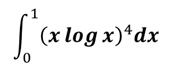3 M
1(b) Solve (D2-1)(D-1)2y=0
3 M
1(c) Prove that E=1+Δ=ehD
3 M
1(d) Solve the following: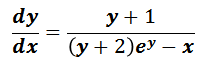3 M
1(e) Change into polar co-ordinates and evaluate: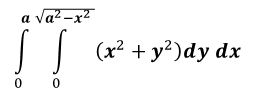4 M
1(f) Evaluate the following: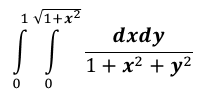4 M

2(a) Solve (x3y3-xy)dy=dx
6 M
2(b) Change the order of Integration and evaluate: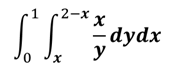6 M
2(c)(1) Prove that: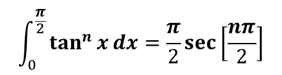4 M
2(c)(2) Evaluate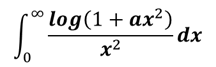, where a>0
4 M

3(a) Evaluate the following: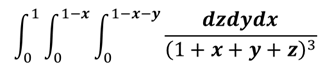6 M
3(b) Find the area bounded by 9xy=4 and 2x+y=2
6 M
3(c)(1) Solve the following: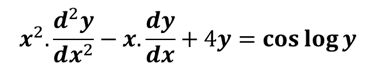4 M
3(c)(2) Solve the equation by variation of parameters: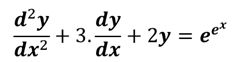4 M

4(a)

Show that for the parabola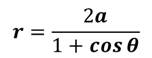from θ=0 to θ=π/2, length of the arc is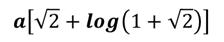6 M
4(b) Solve the following: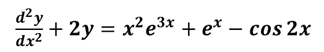6 M
4(c) Apply Runge-Kutta method of fourth order to find an approximation value of y at x=0.2, if dy/dx=x+y2, given y=1 when x=0, in steps of h=0.1
8 M

5(a) Solve: (2xy4ey+2xy3+y)dx+(x2y4ey-x2y2-3x)dy=0
6 M
5(b) Solve dy/dx=2x+y with x0=0,y0=0 by Taylor’s method. Obtain y as a series in power of x. Find approximation value of y for x=0.2,0.4. Compare your result with exact values.
6 M
5(c) Evaluate the following equation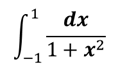by Trapezoidal method, Simpson's 1/3rd and 3/8th methods. Compare result with exact values.
8 M

6(a) In a circuit containing inductance L, resistance R, voltage E, the current i is given by L(di/dt)+Ri=E. Find i at a time t if at t=0,i=0, and if L, R, E are constants.
6 M
6(b) Evaluate ∫∫xy dxdy bounded by y=x, x2+y2-2x=0, and y2=2x
6 M
6(c)(1) Find the volume of a tetrahedron bounded by the plane x=0,y=0,z=0 and x+y+z=a
4 M
6(c)(2) Find the volume bounded by the cone z2=x2+y2 and paraboloid z=x2+y2.
4 M

More question papers from Applied Mathematics 2